You are currently offline. Some features of the site may not work correctly.

# Partial cube

In graph theory, a partial cube is a graph that is an isometric subgraph of a hypercube. In other words, a partial cube is a subgraph of a hypercube… Expand
Wikipedia

## Papers overview

Semantic Scholar uses AI to extract papers important to this topic.
2019
2019
Peano partial cubes are the bipartite graphs whose geodesic interval spaces are (closed) join spaces. They are the partial cubes… Expand
•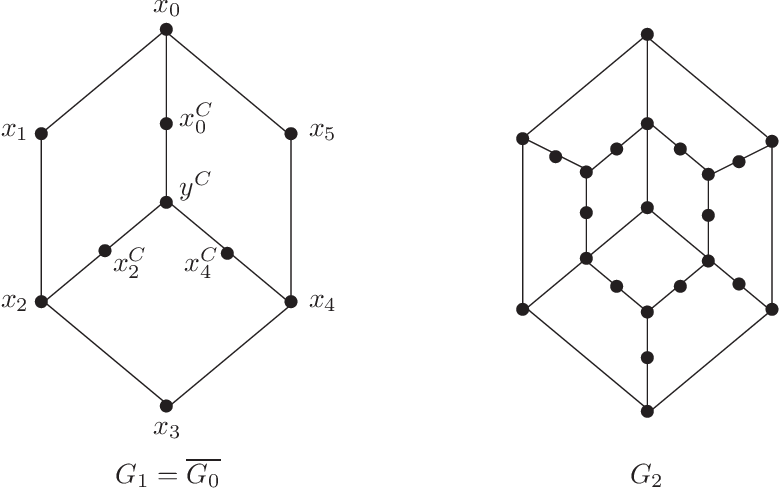•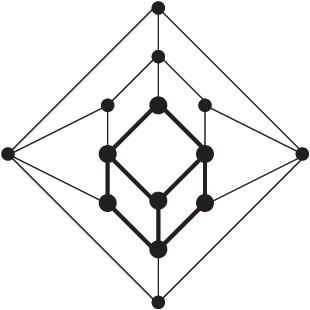•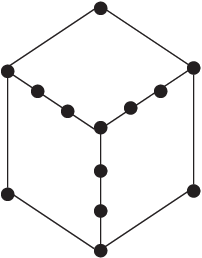•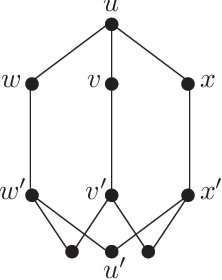•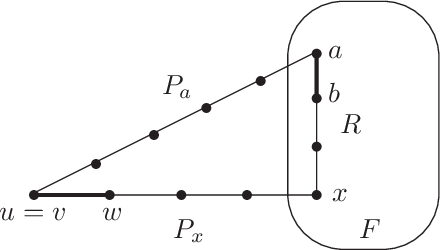Is this relevant?
2017
2017
• Tilen Marc
• Journal of Graph Theory
• 2017
• Corpus ID: 3005803
Partial cubes are graphs isometrically embeddable into hypercubes. In this paper it is proved that every cubic, vertex-transitive… Expand
•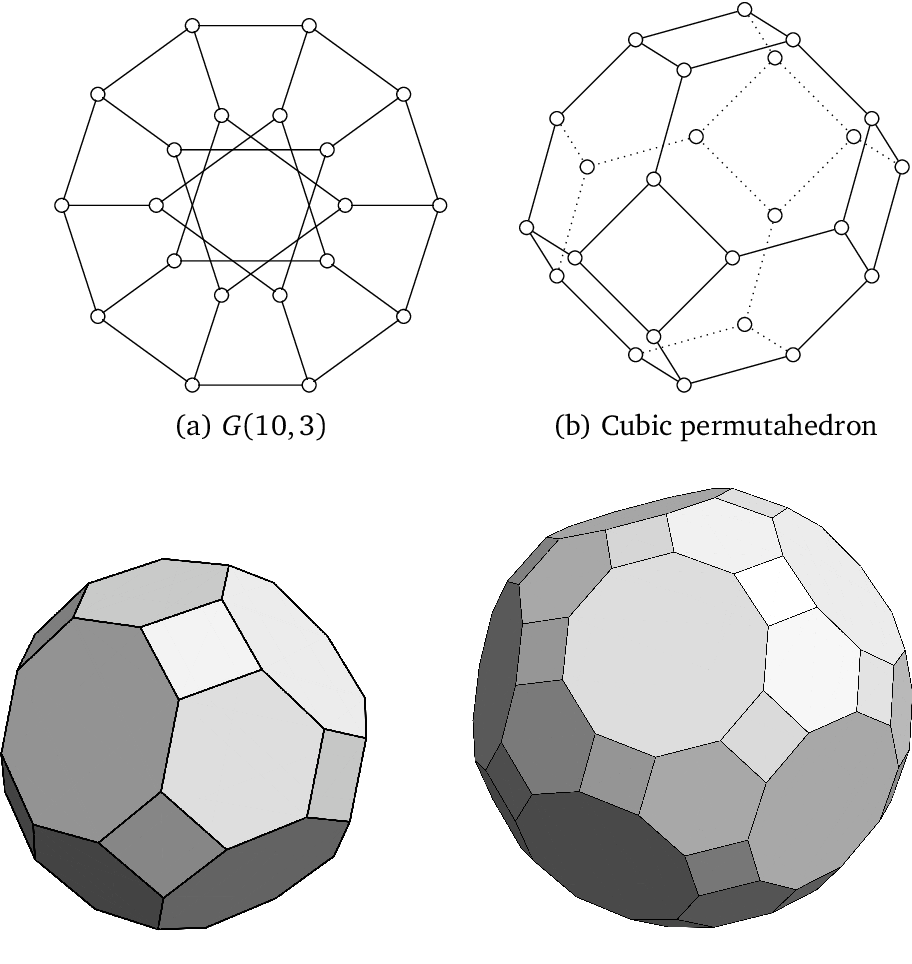•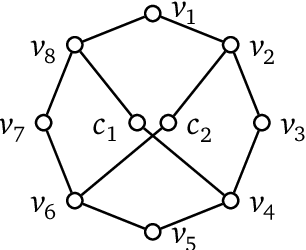•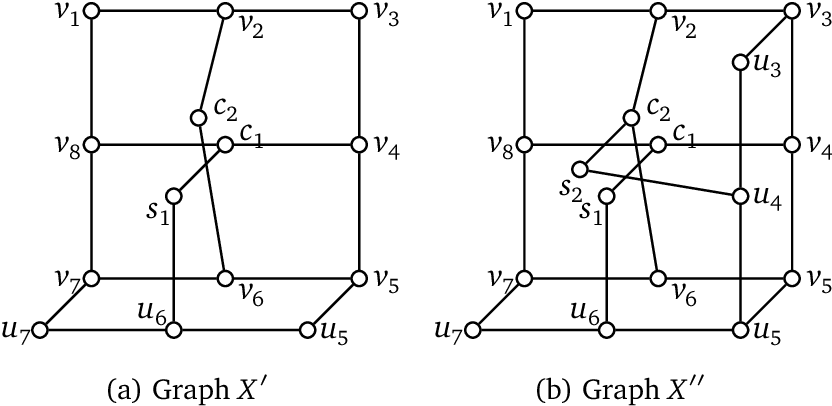•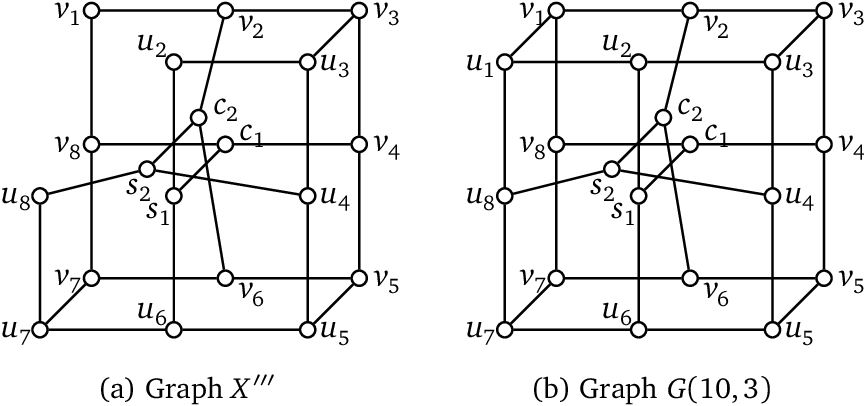•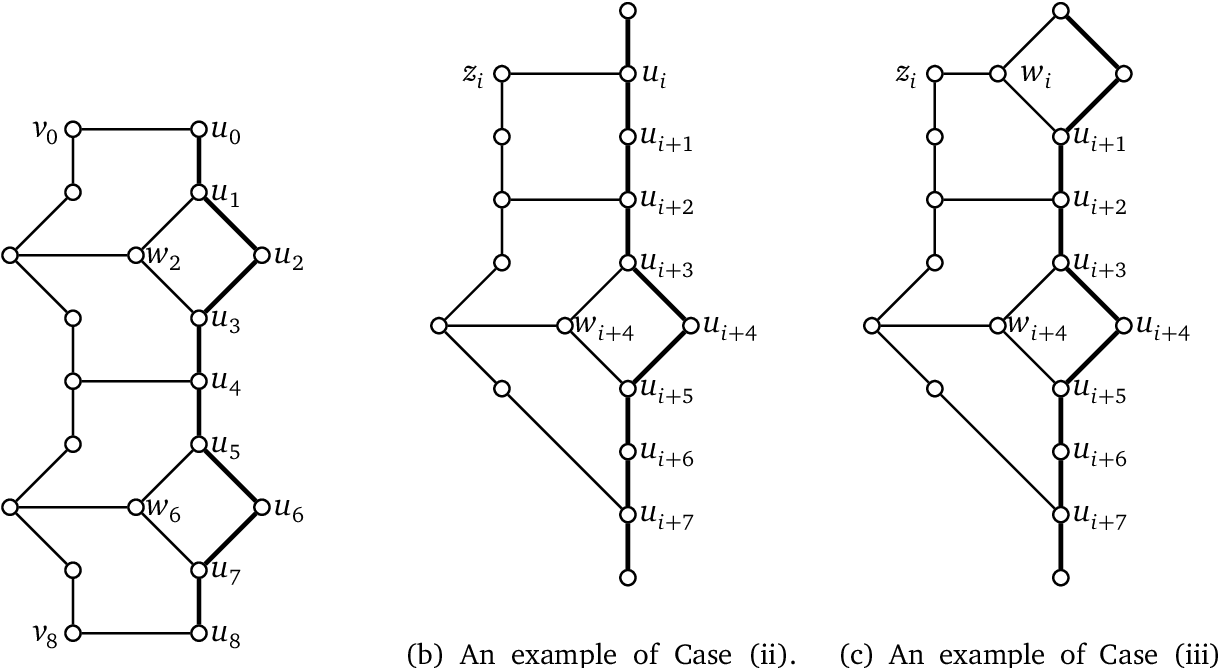Is this relevant?
2016
2016
• ArXiv
• 2016
• Corpus ID: 3016039
We investigate the structure of isometric subgraphs of hypercubes (i.e., partial cubes) which do not contain finite convex… Expand
•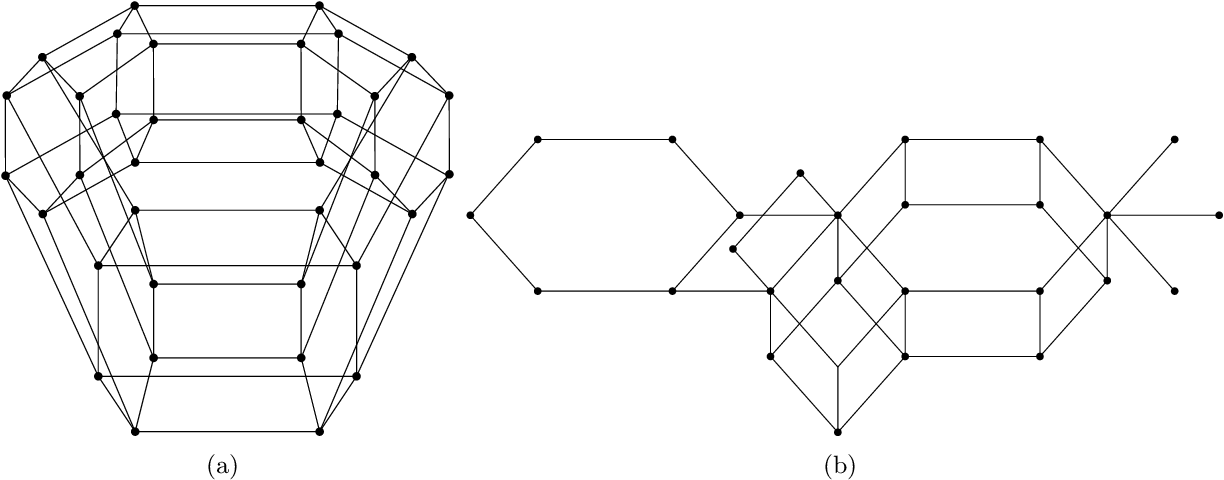•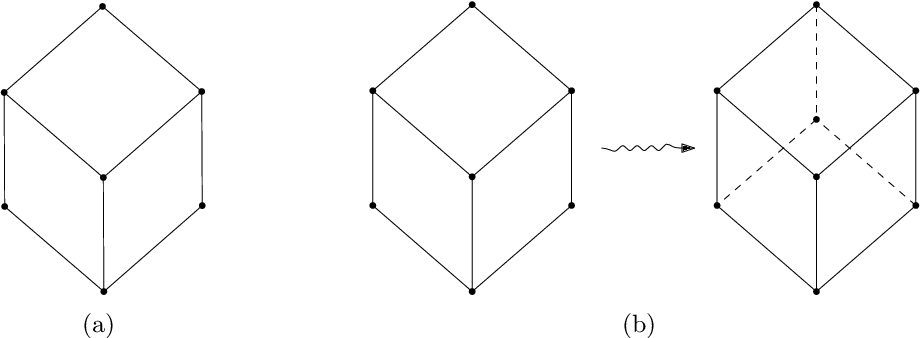•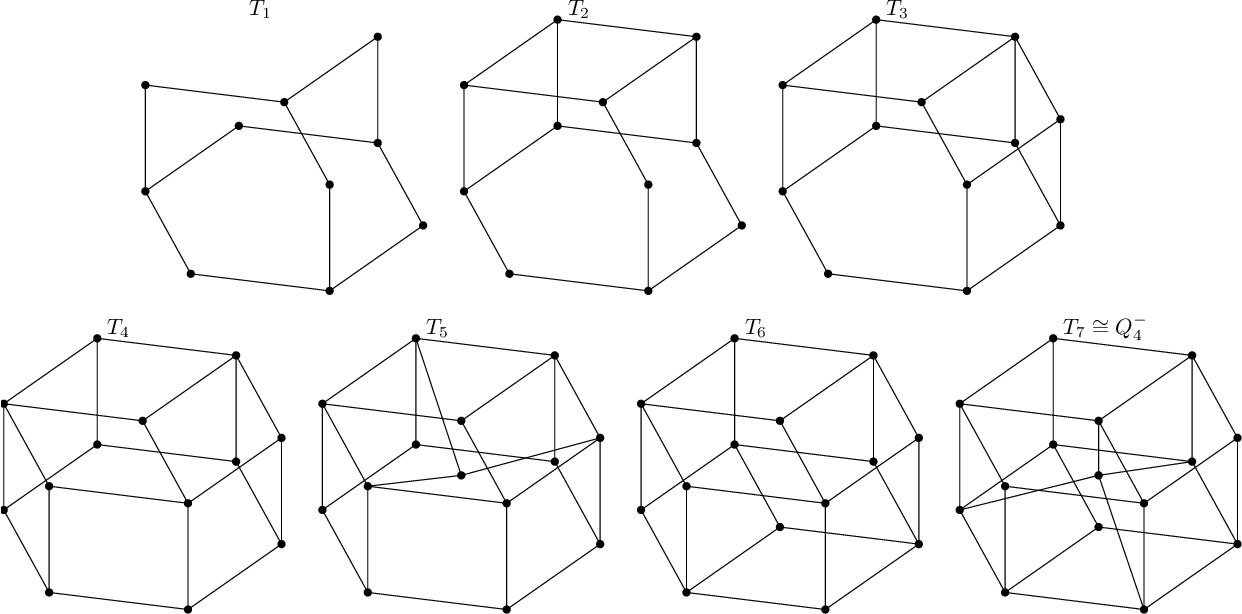•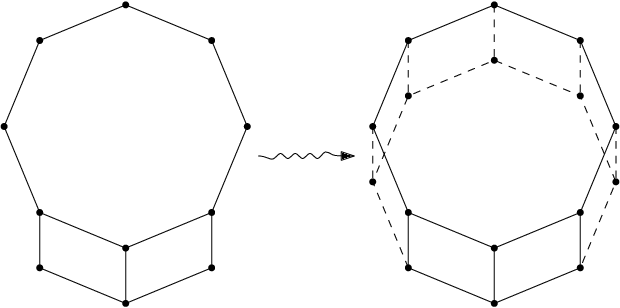•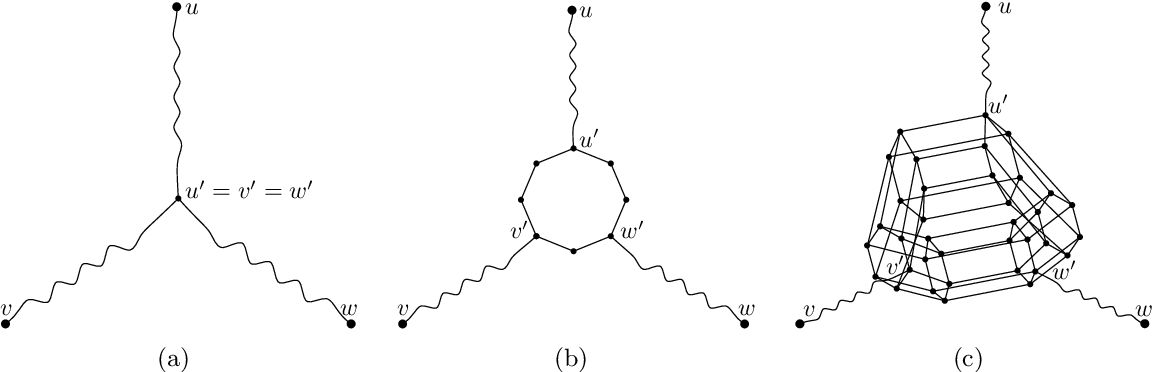Is this relevant?
2012
2012
• Journal of Graph Theory
• 2012
• Corpus ID: 1656835
The convex excess ce(G) of a graph G is introduced as where the summation goes over all convex cycles of G. It is proved that for… Expand
•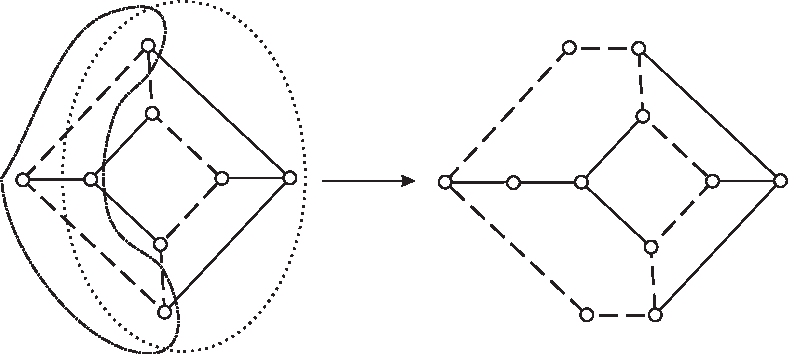•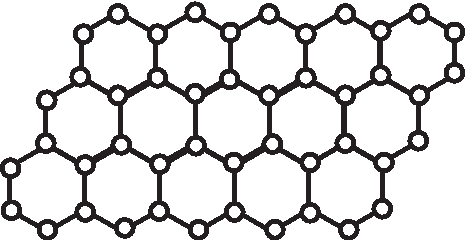Is this relevant?
2009
2009
• Ars Comb.
• 2009
• Corpus ID: 405434
Fukuda and Handa  asked whether every even partial cube G is harmonic-even. It is shown that the answer is positive if the… Expand
•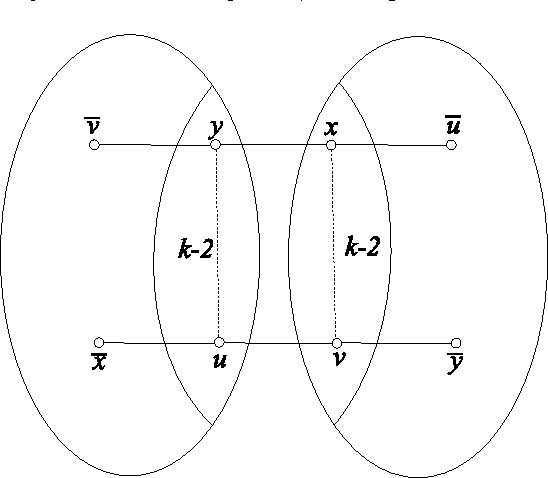•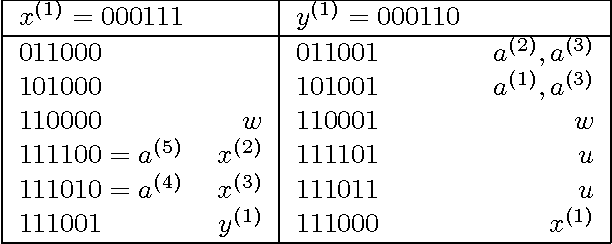Is this relevant?
2009
2009
A graph G has the Median Cycle Property (MCP) if every triple (u"0,u"1,u"2) of vertices of G admits a unique median or a unique… Expand
Is this relevant?
2007
2007
A set A of vertices of a graph G is C-convex if the vertex set of any cycle of the subgraph of G induced by the union of the… Expand
•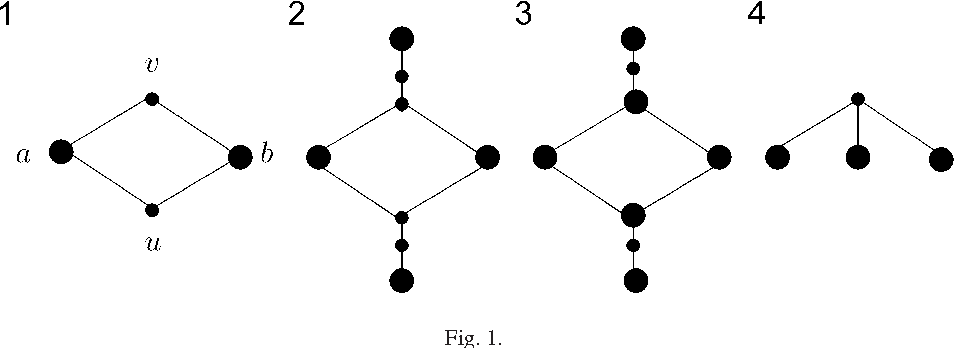•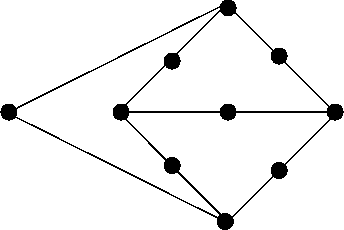•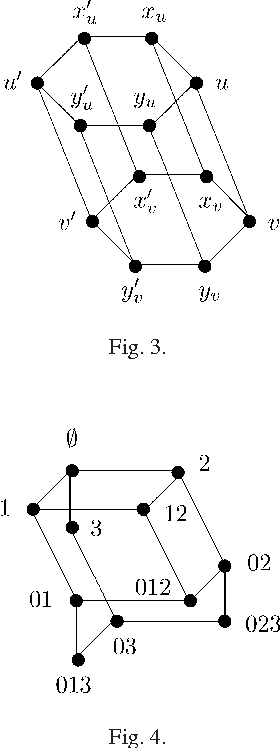•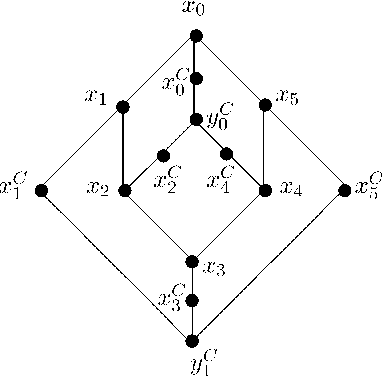•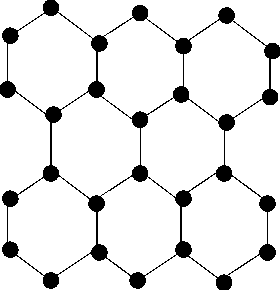Is this relevant?
2003
2003
• Discret. Math.
• 2003
• Corpus ID: 101180
Isometric subgraphs of hypercubes are known as partial cubes. The subdivision graph of a graph G is obtained from G by… Expand
•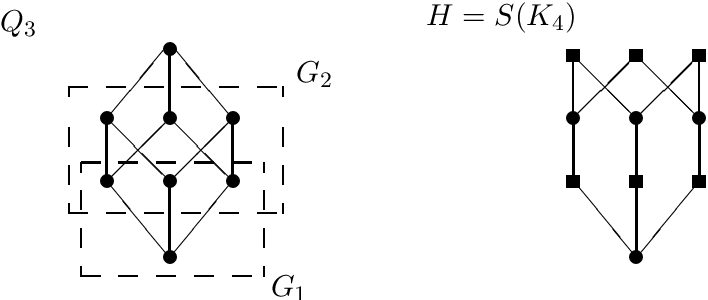•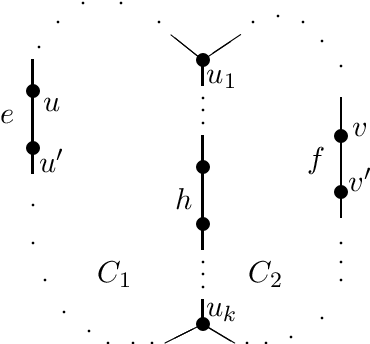•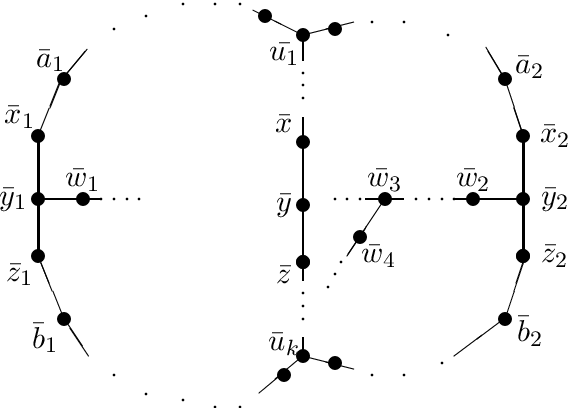•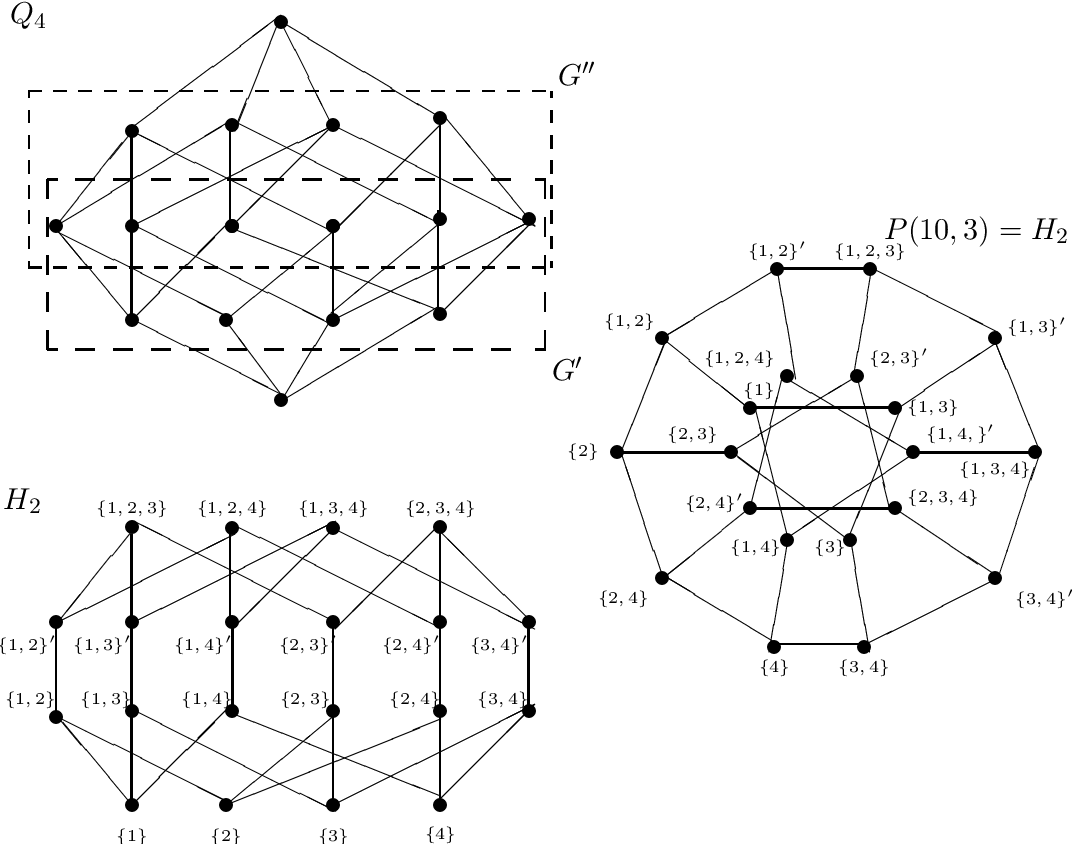Is this relevant?
2002
2002
• SIAM J. Discret. Math.
• 2002
• Corpus ID: 2034411
Partial cubes are defined as isometric subgraphs of hypercubes. For a partial cube G, its crossing graph G# is introduced as the… Expand
•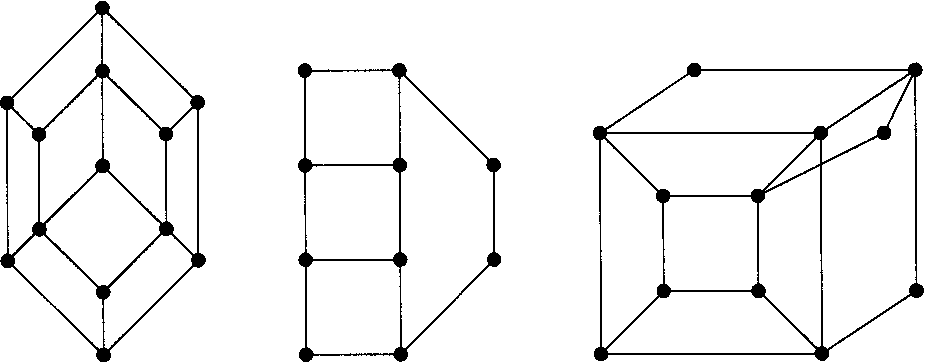•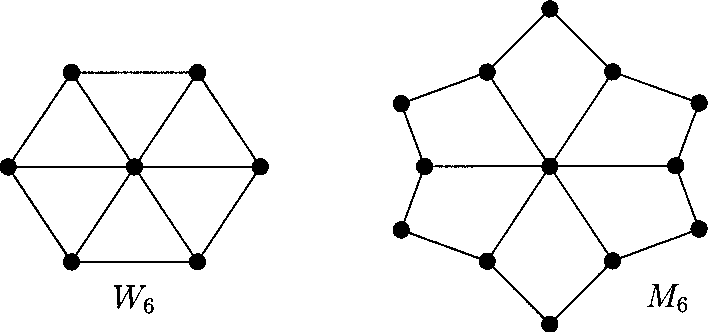•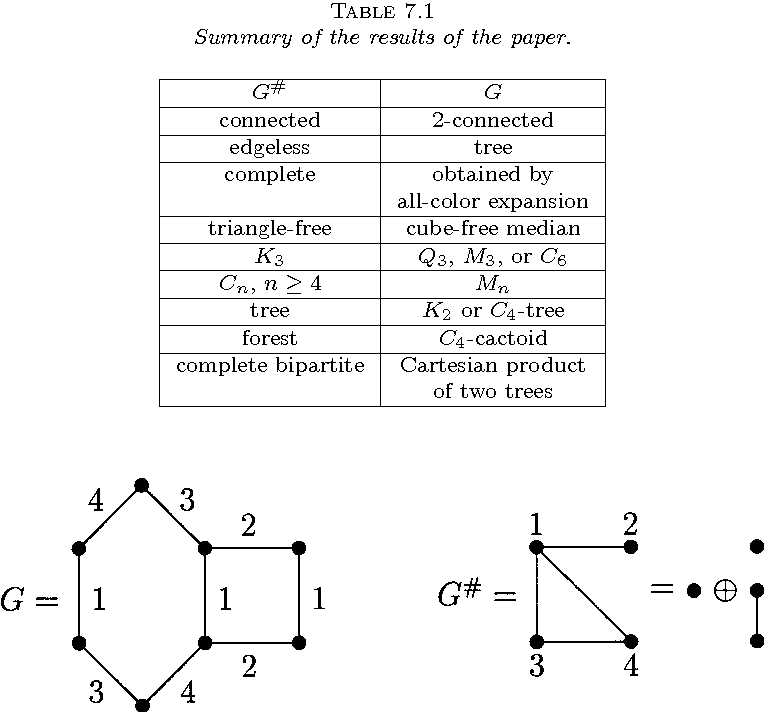•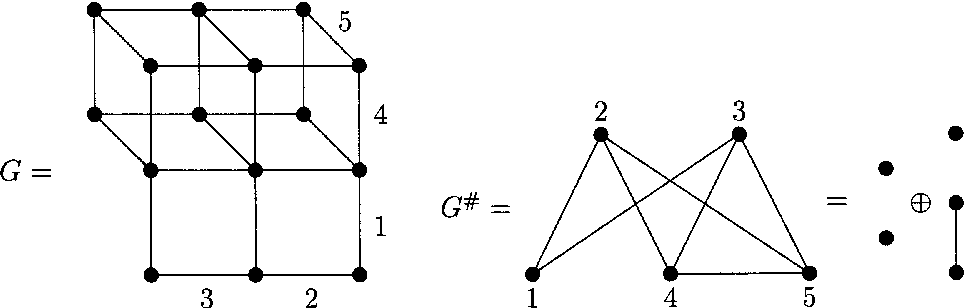Is this relevant?
2002
2002
• Journal of Graph Theory
• 2002
• Corpus ID: 12985830
In the quest to better understand the connection between median graphs, triangle-free graphs and partial cubes, a hierarchy of… Expand
••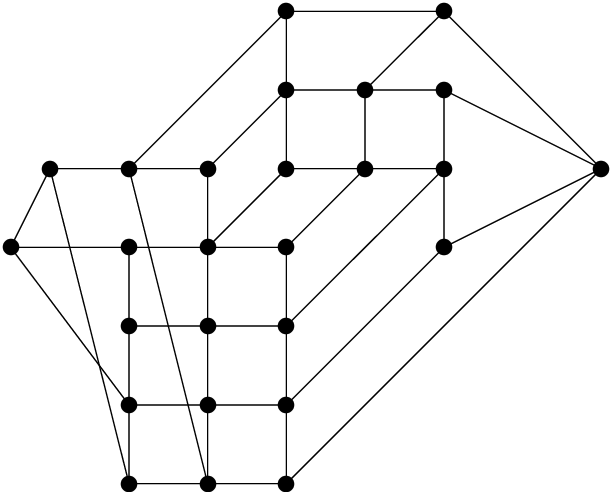•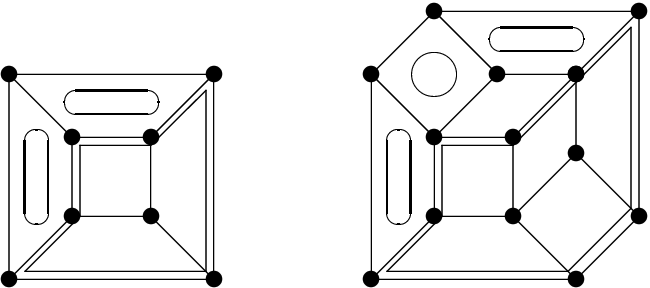Is this relevant?